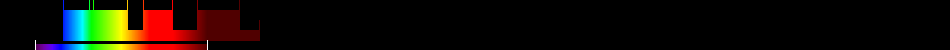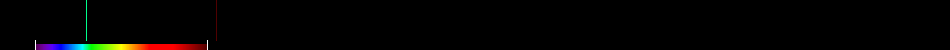# Information for Sensor and Index

## Formula

General Formula $\frac{\frac{{\mathrm{800nm}}}{{\mathrm{500nm}}}}{\frac{{\mathrm{r800}}}{{\mathrm{r500}}}}$ $\frac{\frac{{\mathrm{nahe_Infrarot}}}{{\mathrm{Blau}}}}{\frac{{\mathrm{r800}}}{{\mathrm{r500}}}}$ Automatic

## Sensor

Name IKONOS-2 5 445-900 0.82-3.2 98.1 GeoEye 1999-09-24 original IKONOS-1 was lost during launch at 1999-04-27 yes

## Index

Name Ratio Analysis of Reflectance Spectra C3 RARSc3 $\frac{\frac{{\mathrm{800nm}}}{{\mathrm{500nm}}}}{\frac{{\mathrm{r800}}}{{\mathrm{r500}}}}$ 500,800 Original formula

## References

No results for the selected combination.

## Visualisation

SensorIndex